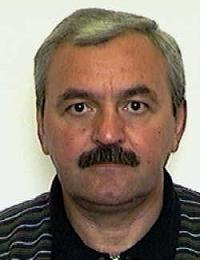Monotone Numerical Methods for Nonlinear Parabolic Problems
Speaker
Prof. Igor Boglaev
Institute of Fundamental Sciences, Massey University, New Zealand
Abstract

The talk is concerned with monotone numerical methods for nonlinear parabolic problems. The basic idea of the iterative methods for the computation of numerical solutions is the monotone approach which involves the notion of upper and lower solutions and the construction of monotone sequences from a suitable linear discrete system. The monotone property of the iterations gives improved upper and lower bounds of the solution in each iteration. The monotone convergence property is used to prove the convergence of the nonlinear discrete problems to the corresponding differential problems as mesh sizes decrease to zero. Applications are given to several models arising from physical, chemical and biological systems. Numerical experiments are given to some of these models, including a discussion on a rate of convergence of the monotone sequences.